Refer to our Texas Go Math Grade 1 Answer Key Pdf to score good marks in the exams. Test yourself by practicing the problems from Texas Go Math Grade 1 Lesson 7.3 Answer Key Use Think Addition to Subtract.

Essential Question
Explanation:
4 + 6 = 10
10 – 6 = 4

Explore
UseDraw to show your work. Write the number sentences.

What is 10 – 3?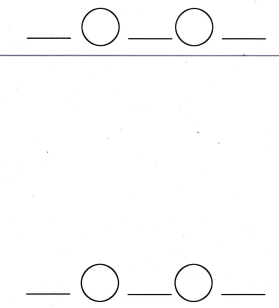Explanation: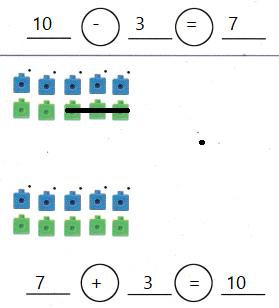10 – 3 = 7
is the subtraction fact
derived a addition fact from it.

For The Teacher

• Read the problem. Maria has 7 games. She gets 3 more. How many games does she have? Children use the top workspace to solve. Then have children solve this problem: Maria has 10 games. She gives 3 of them to her friends. How many games does Maria have now?

Explanation:
7 + 3 = 10
10 games she have now.
10 – 3 = 7
so, she has 7 games now

Math Talk

Mathematical Processes
Explanation:
10 – 3 = 7
and 7 + 3 = 10
so this facts says my answers make sense.

Model and Draw

What is 8 – 6?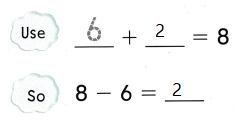Explanation:
An addition fact that  helped to find subtraction fact.

Share and Show

Question 1.
What is 8 – 6?Explanation:
Addition fact helped to find the subtraction fact

Question 2.
What is 11 – 5?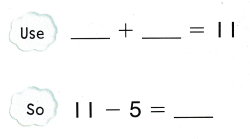Explanation:
Addition fact helped to find the subtraction fact

Question 3.
What is 10 – 8?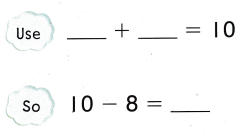Explanation:
Addition fact helped to find the subtraction fact

Question 4.
What is 7 – 4?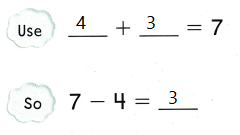Explanation:
Addition fact helped to find the subtraction fact

Problem Solving

Question 5.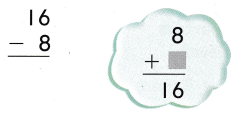Explanation:
Addition fact helped to find the subtraction fact

Question 6.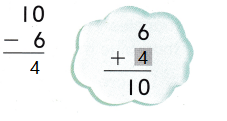Explanation:
Addition fact helped to find the subtraction fact

Question 7.Explanation:
Addition fact helped to find the subtraction fact
7 – 5 = 2
2 + 5 = 7

Question 8.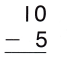Explanation:
Addition fact helped to find the subtraction fact
10 – 5 = 5
5 + 5 = 10

Question 9.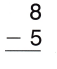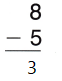Explanation:
Addition fact helped to find the subtraction fact
8 – 5 = 3
3 + 5 = 8

Question 10.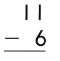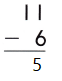Explanation:
Addition fact helped to find the subtraction fact
11 – 6 = 5
5 + 6 = 11

Question 11.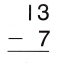Explanation:
Addition fact helped to find the subtraction fact
13 – 7 = 6
6 + 7 = 13

Question 12.
Emil has 13 pencils in a cup. He takes some pencils out. There are 6 pencils left in the cup. How many pencils does he take out?
What addition fact can you use to solve this problem?
___ + ___ = ______So, Emil takes out ______ pencils.
So, Emil takes out 7 pencils.
Explanation:
Emil has 13 pencils in a cup. He takes some pencils out.
There are 6 pencils left in the cup.
13 – 6 = 7
7 pencils that he take out

Question 13.
H.O.T. Multi-Step Mark has 5 red pens and 2 blue pens. He gives 1 blue pen and 1 red pen to Anna. How many pens does he have now?
___ pens
Explanation:
Mark has 5 red pens and 2 blue pens.
5 + 2 = 5
He gives 1 blue pen and 1 red pen to Anna.
1 + 1 = 2
5 – 2 = 3
3 pens that he have now

Question 14.
Rosa has 13 red marbles and 9 blue marbles. How many more red marbles than blue marbles does Rosa have?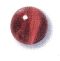(A) 2 more
(B) 3 more
(C) 4 more
Explanation:
Rosa has 13 red marbles and 9 blue marbles.
13 – 9 = 4
4 more red marbles than blue marbles that Rosa have

Question 15.
Analyze Javier is finding 12 – 6. Which addition fact will help him sùbtract?
(A) 6 + 6 = 12
(B) 6 + 4 = 10
(C) 6 + 8 = 14
Explanation:
Analyze Javier is finding 12 – 6.
12 – 6 = 6
6 + 6 = 12 fact will help him subtract

Question 16.
Multi-Step Monique has 14 balloons. She gives 6 balloons to her brother. Then she gives 3 balloons to her sister. How many balloons does Monique have now?(A) 8
(B) 5
(C) 9
Explanation:
Monique has 14 balloons. She gives 6 balloons to her brother.
14 – 6 = 8
Then she gives 3 balloons to her sister.
8 – 3 = 5
5  balloons that Monique have now

Question 17.
Texas Test Prep Which subtraction sentence can you solve by using 3 + 9 = 12?
(A) 9 – 3 = ___
(B) 12 – 6 = __
(C) 12 – 3 = ____
Explanation:
12 – 3 = 9 is the subtraction sentence that can be solved by using 3 + 9 = 12

Take Home Activity

• Ask your child to explain how the addition fact 8 + 6 = 14 can help him or her to find 14 – 6.
Explanation:
8 + 6 = 14
14 – 6 = 8

### Texas Go Math Grade 1 Lesson 7.2 Homework and Practice Answer Key

Question 1.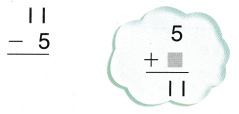Explanation:
Addition fact helped to find the subtraction fact

Question 2.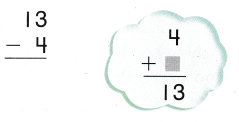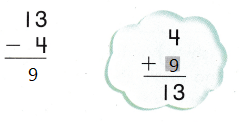Explanation:
Addition fact helped to find the subtraction fact

Question 3.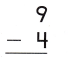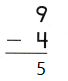Explanation:
Addition fact helped to find the subtraction fact
9 – 4 = 5
5 + 4 = 9

Question 4.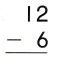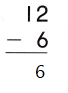Explanation:
Addition fact helped to find the subtraction fact
12 – 6 = 6
6 + 6 = 12

Question 5.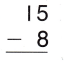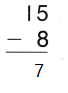Explanation:
Addition fact helped to find the subtraction fact
15 – 8 = 7
8 + 7 = 15

Question 6.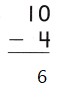Explanation:
Addition fact helped to find the subtraction fact
10 – 4 = 6
6 + 4 = 10

Question 7.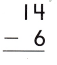Explanation:
Addition fact helped to find the subtraction fact
14 – 6 = 8
6 + 8 = 14

Problem Solving

Question 8.
Leanna invites 15 children to her party. Some children are not able to come. There are 9 children at the party. How many children did not come?
What addition fact can you use to solve this problem?___ + ___ = ____
So, ____ children do not come to the party.
6 + 9 = 15
So, 6 children do not come to the party.
Explanation:
Leanna invites 15 children to her party.
Some children are not able to come.
There are 9 children at the party
15 – 9 = 6
6 children did not come

Lesson Check

Texas Test Prep

Question 9.
Micah wants to find 13 — 7. Which addition fact will help him subtract?
(A) 7 + 5 = 12
(B) 7 + 8 = 15
(C) 7 + 6 = 13

Question 10.
Ashley has II dresses. Because 4 are too small, she gives them away. How many dresses does she have now?
Which addition fact helps you solve the problem?(A) 3 + 8 = 11
(B) 6 + 8 = 14
(C) 4 + 7 = 11

Question 11.
Which subtraction sentence can you solve using 4 + 8 = 12?
(A) 12 – 4 = ___
(B) 12 – 6 = ___
(C) 8 – 4 = ___